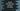# Find the number of days between two dates in Python## Introduction :

Find out the difference between two dates in days using python. For example, if the first date is 2-2-2020 and the second date is 2-3-2020 in day-month-year format, this should print 29 days as the output.

Again, if the first date is 2-3-2020 and the second date is 2-4-2020, it should print _31___

The easiest way to solve it is by using the datetime python module. This module provides different ways to deal with dates. Let’s take a look at the program :

``````from datetime import date

date_one = date(2020, 3, 2)
date_two = date(2020, 4, 2)

difference = date_two - date_one

print(difference)``````

Here,

• we are using date from datetime module.
• dateone_ and datetwo_ are two date objects.
• We are creating these date objects using date(year, month, day) constructor.
• difference variable holds the difference of these two date objects.

This object will print the below output :

``31 days, 0:00:00``

It prints day and time in hour: minute: seconds. If you want to print only the days, use difference.days.